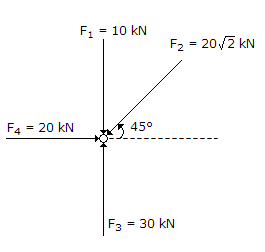# Civil Engineering - UPSC Civil Service Exam Questions

16.

The resultant of the coplanar, concurrent system of forces F1, F2, F3 and F4 shown in the above figure isA. 20kN acting vertically upwards B. zero C. 20 kN acting vertically downwards D. 20 kN acting horizontally from left to right

Explanation:

No answer description available for this question. Let us discuss.

17.

During seepage through an earth mass, the direction of seepage is

 A. parallel to the equipotential lines B. perpendicular to the stream lines C. perpendicular1 to the equipotential lines D. along the direction of gravity

Explanation:

No answer description available for this question. Let us discuss.

18.

Consider the following statements :
The effect of sea water on hardened concrete is to
1. increase its strength
2. reduce its strength
3. retard setting
4. decrease its durability.
Of these statements :

 A. 1 and 3 are correct B. 2 and 3 are correct C. 2 and 4 are correct D. 1 and 4 are correct

Explanation:

No answer description available for this question. Let us discuss.

19.

A doubly reinforced concrete beam has effective cover d' to the centre of compression reinforcement. xu is the depth of neutral axis, and d is the effective depth to the centre of tension reinforcement. What is the maximum strain in concrete at the level of compression reinforcement?

 A. 0.0035 (1 - d'/d) B. 0.0035(1 - d'/xu) C. 0.002(1 - d'/xu) D. 0.002(1 - d'/d)

Explanation:

No answer description available for this question. Let us discuss.

20.

By what percentage is length of a runway increased for every 300 m rise above M.S.L. ?

 A. 3% B. 4% C. 6% D. 7%